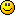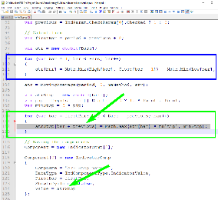#### Topic: Use previous bar value (UPBV) question

I have noticed 2 different versions of indicators by C++ code:
1) Indicator [bar] = Function of O, H, L, C [bar-1, bar-2, bar-3…..]
2) Indicator [bar] = Function of O,H, L, C [bar, bar-1, bar-2, bar-3,...]

Question:

If we use UPBV=true and indicator 1): will the result be shifted by 2 bars ? (1 bar in C++ code and 1 bar by means of UPBV = true)

#### Re: Use previous bar value (UPBV) question

It is C#, not C++Can you give a real example from the indicators? It would help to explain better.

Secondly, previous bar value comes in to play in the logic calculation, where trading signals are raised. In some custom indis you might find PBV cut out and taken into account already in the indi value calculation block.

Thirdly, if only open prices are used in the calculation, PBV is not used, in FSB PBV is automatically switched off. If, for example, there are other price points used in addition (or without open prices), PBV is a must.

Taking into account those points above, in your abstract example case nr 1 doesn't seem to need the use of PBV, but the 2nd case needs it.

Finally, I would not describe it as a "shift". It is extremely vital necessity for having a reliable and reproducible backtest result. It is a matter of using the latest confirmed datapoints! It doesn't shift, but uses latest closed values, which do not change.

#### Re: Use previous bar value (UPBV) question

In addition to Footon's explanations, I may add that there is a difference between the values of the plotted line and the actual signals. The indicators show and plots their lines without PBV in order to look like as the MetaTrader indicators in the chart. The PBV is used when the indicator calculates the trading signals.

#### Re: Use previous bar value (UPBV) question

footon wrote:

It is C#, not C++Can you give a real example from the indicators? It would help to explain better.

Secondly, previous bar value comes in to play in the logic calculation, where trading signals are raised. In some custom indis you might find PBV cut out and taken into account already in the indi value calculation block.

Thirdly, if only open prices are used in the calculation, PBV is not used, in FSB PBV is automatically switched off. If, for example, there are other price points used in addition (or without open prices), PBV is a must.

Taking into account those points above, in your abstract example case nr 1 doesn't seem to need the use of PBV, but the 2nd case needs it.

Finally, I would not describe it as a "shift". It is extremely vital necessity for having a reliable and reproducible backtest result. It is a matter of using the latest confirmed datapoints! It doesn't shift, but uses latest closed values, which do not change.

Example of indicator 1) - Pinbar M (uses only Bar-1 values):
for (int iBar = 1; iBar < Bars; iBar++)
{
if (Math.Abs(Close[iBar - 1] - Open[iBar - 1]) < iPERCENT * 0.01 * (High[iBar - 1] - Low[iBar - 1]))
doji[iBar] = 1;

Example of indicator 2) - ATR (AverageTrueRange) - (uses High[bar], Low[bar]):
for (int bar = 1; bar < Bars; bar++)
{
atr[bar] = Math.Max(High[bar], Close[bar - 1]) - Math.Min(Low[bar], Close[bar - 1]);
}

atr = MovingAverage(period, 0, maMethod, atr);

#### Re: Use previous bar value (UPBV) question

As I said before, some indicators apply the PBV later, when they actually calculate the signals. If you see the ATRStop indicator, it first calculates ATR and then uses the PBV when calculates the signal array `atrStop`.#### Re: Use previous bar value (UPBV) question

Yes, Pinbar M is mine. The setting of PBV you see in FSB affects it in no way. Signal bar is not shifted, neither it is delayed. It uses closed bars, which value is known. And therefore it calculates and works reliably.